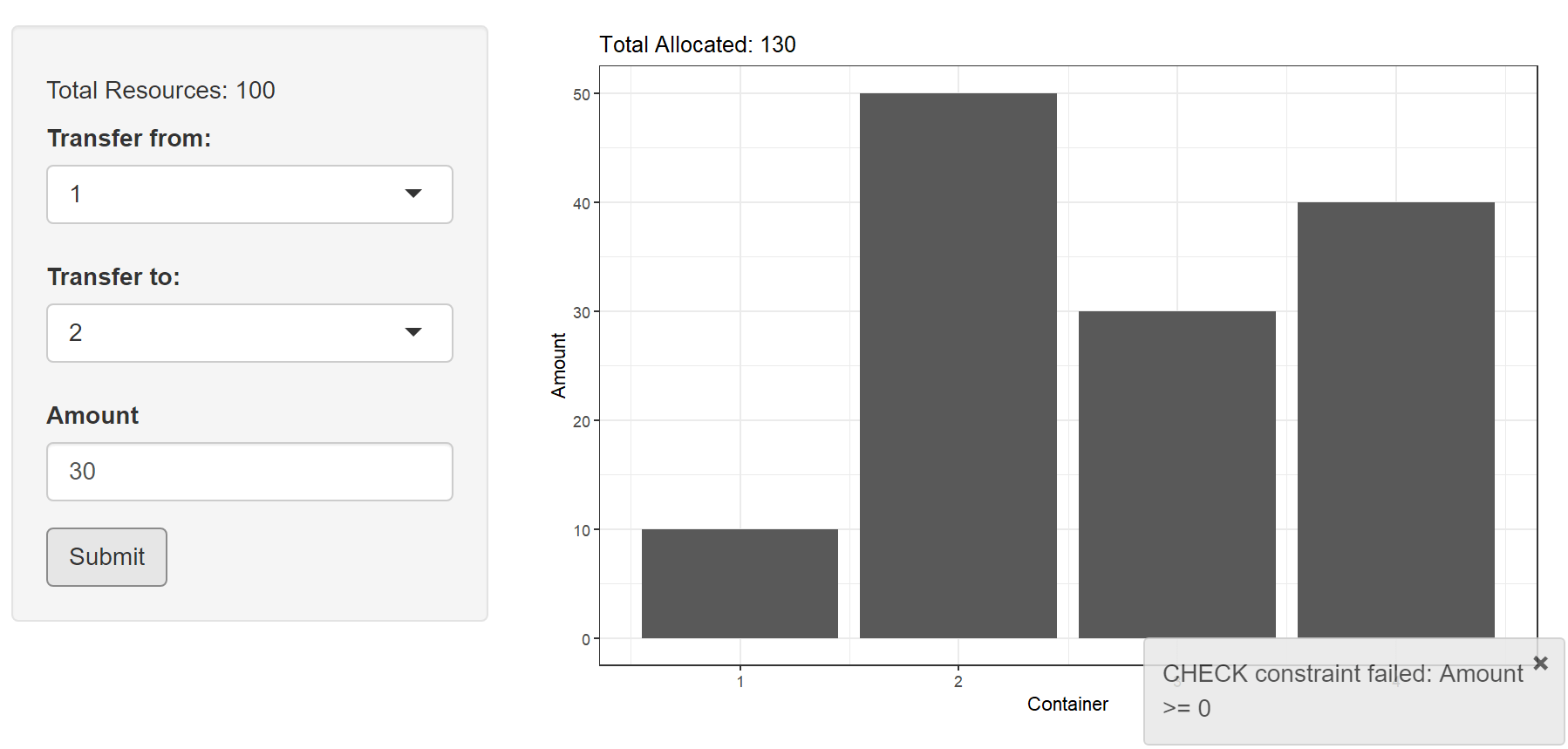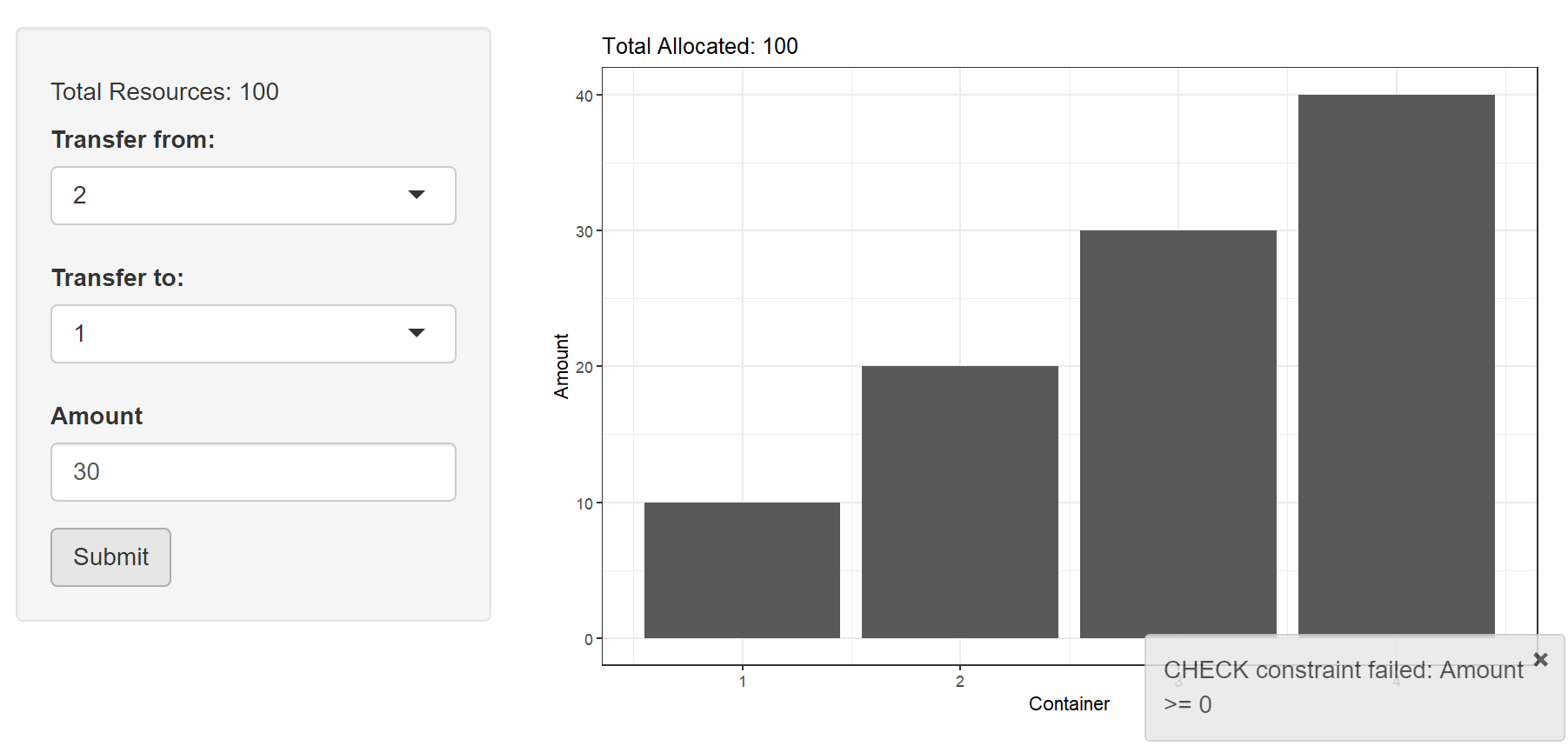[This article was first published on R on Thomas Roh, and kindly contributed to R-bloggers]. (You can report issue about the content on this page here)
Want to share your content on R-bloggers? click here if you have a blog, or here if you don't.

An important aspect of maintaining databases with an application interface is to ensure atomicity. When performing multiple writes on a database, any failures that occur during the operations should not violate any logical rules. The most common analogy is a financial transaction. If person A withdraws X dollars from their account and deposits into the account of person B then the withdrawal and deposit should occur as a whole transaction. If only one occurs, you have either created or deleted currency (your government does not approve of this). The `DBI` package provides a couple of abstractions to perform transactions with R and seamlessly implement them into a `shiny` application.

The following demonstrates maintaining atomicity through an application that allows the user to re-allocate resources to 4 containers. Any container can transfer resources to another container but the amount must be greater than 0 and less than what is currently in the container that is losing resources. The condition that should always be met is that the sum of the resources should equal 100. The full application is at the end of the post for reference.## Create a Database

For the example, a database is created with a table that stores the amount of resources in each container. The total amount of resources available is limited to 100 and is pre-populated with initial values. A constraint has been placed that will be important later on.

```library(DBI)
library(RSQLite)
con <- dbConnect(RSQLite::SQLite(), "allocations.sqlite")
dbExecute(con,
'CREATE TABLE Containers (Container int, Amount float, CHECK(Amount >= 0))'
)
dbExecute(con, 'INSERT INTO Containers (Container, Amount) VALUES(1, 10)')
dbExecute(con, 'INSERT INTO Containers (Container, Amount) VALUES(2, 20)')
dbExecute(con, 'INSERT INTO Containers (Container, Amount) VALUES(3, 30)')
dbExecute(con, 'INSERT INTO Containers (Container, Amount) VALUES(4, 40)')
dbGetQuery(con, 'SELECT Container, Amount FROM Containers')
```## Re-allocate Resources with Independent SQL Statements

The simplest approach is to execute two statements on the table in series. Using some server-side logic you can control that minimum and maximum amounts (roll your own logic with if/else statements and `showNotification` or `showModal` or check out shinyvalidate). As long as both of these statements execute, the application will perform as intended.

```dbExecute(
con,
sqlInterpolate(
con,
'UPDATE Containers SET Amount = Amount + ?TransferAmount
WHERE Container = ?ToContainer',
TransferAmount = input\$Amount,
ToContainer = input\$To
)
)
dbExecute(
con,
sqlInterpolate(
con,
'UPDATE Containers SET Amount = Amount - ?TransferAmount
WHERE Container = ?FromContainer',
TransferAmount = input\$Amount,
FromContainer = input\$From
)
)
```Because the database and application interactions are simple, the chance of an error occuring is relatively small (maybe not that small, network/connectivity errors are common enough). Depending on the use case, you may want to put some calculations in between those two statements, create more complicated database interactions, or execute some side effects. Any additions increase the risk of failure which can lead to results such as this:The first statement executed but the second one that would balance the resources was never run due to an error. There are some ways to handle this on the database side which would prevent data loss (different topic and can be extremely complicated). To handle this exception, when one statement fails all statements should fail. This is the concept of a transaction and can be handled on the application side in `shiny`.

## Re-allocate Resources with a Transaction

Start a transaction with `dbBegin` that will wrap the following database interactions into a single statement. There are three possible outcomes to close the transaction: `dbCommit` will make the changes, `dbRollback` will cancel any changes, and any closing of the connection will also cancel the changes. `tryCatch` is used to handle the errors cleanly (an error will crash the application) and you can also use the error message to alert the user what went wrong.

```tryCatch({
dbBegin(con)
dbExecute(
con,
sqlInterpolate(
con,
'UPDATE Containers SET Amount = Amount + ?TransferAmount
WHERE Container = ?ToContainer',
TransferAmount = input\$Amount,
ToContainer = input\$To
)
)
dbExecute(
con,
sqlInterpolate(
con,
'UPDATE Containers SET Amount = Amount - ?TransferAmount
WHERE Container = ?FromContainer',
TransferAmount = input\$Amount,
FromContainer = input\$From
)
)
dbCommit(con)
}, error = function(e) {
dbRollback(con)
e
})
```## Handling Invalid Values

Now the user can enter invalid values and we can safely prevent them from putting the database into an undesirable state. We can rely on the database to alert the user with the error (that is the `CHECK` constraint that was placed during creation of the database). You can also handle the check on the application side with input validation which works with a single user, but can trip up concurrent users when transactions happen simultaneously or close enough together that the client side values in shiny are not up to date–isolation is a separate part of ACID.

## Full Code

```library(shiny)
library(ggplot2)
library(DBI)
library(RSQLite)
make_reactive_trigger <- function() {
rv <- reactiveValues(a = 0)
list(
depend = function() {
rv\$a
invisible()
},
trigger = function() {
rv\$a <- isolate(rv\$a + 1)
}
)
}
DBTrigger <- make_reactive_trigger()
con <- dbConnect(RSQLite::SQLite(), "allocations.sqlite")
dbExecute(con,
'CREATE TABLE Containers (Container int, Amount float, CHECK(Amount >= 0))')
dbExecute(con, 'INSERT INTO Containers (Container, Amount) VALUES(1, 10)')
dbExecute(con, 'INSERT INTO Containers (Container, Amount) VALUES(2, 20)')
dbExecute(con, 'INSERT INTO Containers (Container, Amount) VALUES(3, 30)')
dbExecute(con, 'INSERT INTO Containers (Container, Amount) VALUES(4, 40)')
initContainers <- dbGetQuery(con, 'SELECT Container, Amount FROM Containers')

ui <- fluidPage(

sidebarLayout(
sidebarPanel(
tags\$h5('Total Resources: 100'),
selectInput('From', 'Transfer from:',
choices = initContainers[['Container']]),
selectInput('To', 'Transfer to:',
choices = initContainers[['Container']][-1]),
numericInput('Amount', 'Amount', min = 0, value = 0,
max = initContainers[['Amount']]),
actionButton('Submit', 'Submit')
),
mainPanel(
plotOutput("AllocationPlot")
)
)
)

server <- function(input, output, session) {
Allocations <- reactive({
DBTrigger\$depend()
dbGetQuery(con, 'SELECT Container, Amount FROM Containers')
})
#  Transferring to self is redundant
observeEvent(input\$From, {
session,
'To',
choices = Allocations()[['Container']][
!Allocations()[['Container']] %in% input\$From])
})
# Limit tranfer amount
# this will produce a popup warning but is not currently enforced
# server side
# see shinyvalidate package if using bootstrap3
observeEvent(Allocations(), {
i <- Allocations()[['Container']] == input\$From
updateNumericInput(
session, 'Amount',
max = Allocations()[['Amount']][i])
})
observeEvent(input\$Submit, {
res <- tryCatch({
dbBegin(con)
# disallow using negative values
stopifnot(input\$Amount > 0)
dbExecute(con,
sqlInterpolate(con,
'UPDATE Containers
SET Amount = Amount + ?TransferAmount
WHERE Container = ?ToContainer',
TransferAmount = input\$Amount,
ToContainer = input\$To)
)
dbExecute(con,
sqlInterpolate(con,
'UPDATE Containers
SET Amount = Amount - ?TransferAmount
WHERE Container = ?FromContainer',
TransferAmount = input\$Amount,
FromContainer = input\$From)
)
dbCommit(con)
}, error = function(e) {
dbRollback(con)
e
})
if ( inherits(res, 'error') ) {
}
DBTrigger\$trigger()
})
# Show Latest Allocations
output\$AllocationPlot <- renderPlot({
ggplot(Allocations()) +
geom_col(aes(Container, Amount)) +
labs(title = sprintf('Total Allocated: %d',
sum(Allocations()[['Amount']]))) +
theme_bw()
})
}
shinyApp(ui = ui, server = server)
```

To leave a comment for the author, please follow the link and comment on their blog: R on Thomas Roh.

R-bloggers.com offers daily e-mail updates about R news and tutorials about learning R and many other topics. Click here if you're looking to post or find an R/data-science job.
Want to share your content on R-bloggers? click here if you have a blog, or here if you don't.

# Never miss an update! Subscribe to R-bloggers to receive e-mails with the latest R posts.(You will not see this message again.)

Click here to close (This popup will not appear again)